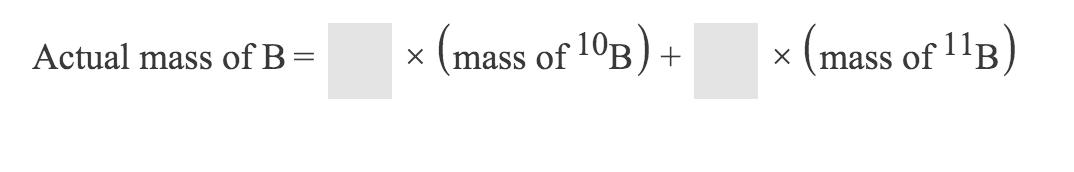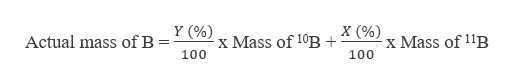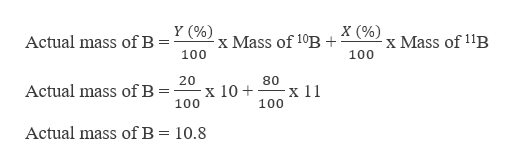Question
7 views

Boron consists of two isotopes. The reported mass of boron is the weighted average of these two isotopes.

Part 1: Complete the equation below that shows how to calculate the actual mass of a boron atom using x for the abundance of B-11 and y for the abundance of B-10.

Part 2: If x and are the fractional abundances of each isotope, then what must x and y add up to?

x+y=?help_outlineImage TranscriptioncloseActual mass of B = mass of 10B mass ofB X fullscreen
check_circle

Step 1

Boron has two isotopes which are B-10 and B-11. It is given that the abundance of B-11 is x and for B-10 is y. The abundance is generally expressed in terms of percentage and hence the actual mass of boron can be calculated as,help_outlineImage TranscriptioncloseY (%) x Mass of 10B + 100 Actual mass of B x Mass of 1B 100 fullscreen
Step 2

In nature, the abundance of B-10 is around 20 % whereas for B-11 is 80%. Hence if we substitute (...help_outlineImage TranscriptioncloseY (% x Mass of 10B +^ xMass of 11B Actual mass of B 100 100 20 80 x 10 100 Actual mass of B x 11 100 Actual mass of B 10.8 fullscreen

### Want to see the full answer?

See Solution

#### Want to see this answer and more?

Solutions are written by subject experts who are available 24/7. Questions are typically answered within 1 hour.*

See Solution
*Response times may vary by subject and question.
Tagged in
ScienceChemistry

### General Chemistry